# Analytical and Numerical Solution of Viscous Fluid Flow with the Effects of Thermal Radiation and Chemical Reaction Past a Vertical Porous Surface

Analytical and Numerical Solution of Viscous Fluid Flow with the Effects of Thermal Radiation and Chemical Reaction Past a Vertical Porous Surface

Partha Protim Gharami Shikdar M. Arifuzzaman Sheikh Reza-E-Rabbi Mohammad Shakhaoath KhanSarder Firoz Ahmmed

Mathematics Discipline, Khulna University, Khulna 9208, Bangladesh

Centre for Infrastructure Engineering, Western Sydney University, NSW 2751, Australia

Department of Basic Sciences and Humanities, University of Asia Pacific, Dhaka 1207, Bangladesh

Department of Chemical Engineering, Monash University, Clayton, VIC, 3800, Australia

Corresponding Author Email:
ms.khan@monash.edu
Page:
689-700
|
DOI:
https://doi.org/10.18280/ijht.380313
27 July 2019
|
Accepted:
2 March 2020
|
Published:
15 October 2020
| Citation

OPEN ACCESS

Abstract:

Magnetohydrodynamic (MHD) unsteady flow of incompressible, electrically conducting, and viscous fluid passing through a vertical porous plate is explored in this investigation where the impact of thermal radiation and chemical reaction are presented. A nonlinear two-dimensional unsteady flow model is created to decompose heat and mass transfer of this fluid, which is shaped by taking appropriate boundary conditions. The governing equations are solved analytically with satisfactory boundary conditions by using a two-term perturbation technique. The analytical outcomes are illustrated graphically by using MATHEMATICA 7.0. The fundamental equations are also solved numerically by utilizing explicit finite difference methodology (EFDM) with the help of Compaq Visual Fortran 6.6a. The numerical arrangement is also depicted graphically to examine the outcomes. The acquired pertinent parameters are analysed conveniently, including thermal Grashof number, thermal radiation parameter, and chemical reaction parameter, the permeability of the porous medium, magnetic parameter, Prandtl number, modified Grashof number, and Schmidt number. It is observed from our experiment that the velocity and concentric profiles decline when the reaction rate between the molecules is high. Furthermore, a suitable validation has been done with the previously published articles, and a favorable agreement is observed.

Keywords:

permeability, perturbation, EFDM, nanoparticles, radiation absorption, chemical reaction, thermal radiation, stability and convergence test

1. Introduction

These days with respect to various expanding applications in material procedure, synthetic and mechanical building, the investigation of liquid elements through permeable channel has been exceptionally well known in research zone. As a result of its conceivable solicitation to geophysical learning and astrophysical learning, free convective stream in nearness of Thermal source has turned into a subject of enthusiasm of numerous scientists. This kind of streams emerge either because of limit temperature or precarious movement of the limit. In numerous industry and numerous other mechanical fields, the investigation of fluctuating stream is essential. Thusly, numerous analysts have given their consideration towards magneto hydrodynamics rivulet of sticky, incompressible liquid past a penetrable channel. It is viewed as that such sort of liquids are suspension, cleanser, paints, restorative, oil, custard, coal, oil slurries, mud covering and physiological fluids (blood, bile and synovial liquid). The issue of free convective Thermal and mass barter limit layer stream with synthetic response and Thermal radiation is critical in present time of current science, innovation and wide extending industrialization. Heat generation, production of sustenance, polymer expulsion in a dissolve turning process, glass fiber and streamlined expulsion of plastic sheets are the building utilizations of the limit layer conduct of viscoelastic liquid. Consolidated Thermal and mass barter with matter response in geometric with and without permeable media have been contemplated by creators. Common convection coming about because of the consolidated Thermal and mass buoyance powers has gotten impressive consideration of designers and researchers because of numerous applications in building and innovative procedures. Many building forms happen at high temperature, in the structure of gear the information of radiative Thermal exchange assumes noteworthy job.

The study is undertaking to explore the fluid flow through an infinite vertical penetrable plate with the effect of thermal radiation, MHD, and chemical reaction on heat and mass transfer. The boundary conditions and mathematical models are settled by utilizing analytically and numerically with the help of perturbation and EFDM. Stability and convergence analysis has been performed for the accuracy of the numerical parameter. The parameters, including magnetic parameter, thermal Grashof number, thermal radiation, Prandtl number, modified Grashof number, Schmidt number, and porous parameter, have been estimated respectively. The productive outcome and comparison have been shown in results section with graphically and also with visualisation of fluid through streamlines and isotherm-lines.

2. Mathematical Formulation

In this paper, it is viewed as two dimensional temperamental MHD free convective progressions of incompressible schmaltzy and electrically conducting liquid affected by intensity and fixation buoyance impacts which are gone through a semi-unending plumb heated absorbent sheet trimmed in a penetrable medium. Between the diffusing species and the liquid there exist a first-order homogenous substance response $\bar{K} r$ with steady rate which is assumed in this paper. It is also assumed that in upward direction along with the plate $\bar{x}$ be taken vertically and $\bar{y}$ be regard as normal to the plate. Toward the path opposite to the sheet a identical magnetic field is connected. In the energy equation viscous attenuation and the joule temperature effect are considered to be ignorable. In case of no applied voltage, the electric field vanishes. It is considered very small magnetic parameter Reynolds number and transvers applied magnetics parameter field, so that the produced magnetic region is negligible. In the parallel amalgamation of the centralization of the diffusing species is thought to be little in examination with the other chemical species type, which are exist. The temperature in the fluid flow is controlled by the energy and concentration equation where the Soret and Dufour effects are unconsidered.

The parameters are considered in this paper such as $\bar{u}, \bar{v}$ are the velocity components in $\bar{x}, \bar{y}$ direction respectively and $\bar{T}, \bar{T}_{\infty} \bar{C}, \bar{C}_{\infty}$ denotes boundary layer temperature, fluid temperature faraway out of the sheet, boundary layer concentration, concentration faraway out of the surface respectively and $\beta, \bar{\beta}$ indicates the expansion coefficient due to temperature and expansion coefficient due to concentration and $\bar{t}, \bar{p}, \mathrm{k}, \rho, \mathrm{g}, \bar{K}, \mathrm{v}, \alpha$ denotes time period, external pressure, Thermal conductivity, fluid density, acceleration for gravitational force, permeability of the perforated medium, kinematic viscosity, fluid Thermal diffusivity, $\sigma$-electric conductivity, Bo-magnetic induction, $\bar{q}_{r}$-radioactive heat flux, $C_{p}$-specific heat when pressure is constant, D-chemical molecular diffusivity and $\bar{K} r$- chemical reaction.

Then ruling equations of fluid which are conservation of mass i.e. continuity, momentum, energy and concentration equations can be expressed as:

Continuity Equation:

$\frac{\partial \bar{v}}{\partial \bar{y}}=0$      (1)

Momentum Equation:

$\frac{\partial \bar{u}}{\partial \bar{t}}+\bar{v} \frac{\partial \bar{u}}{\partial \bar{y}}=v \frac{\partial^{2} \bar{u}}{\partial \bar{y}^{2}}+g \beta\left(\bar{T}-\bar{T}_{\infty}\right)+g \beta\left(\bar{C}-\bar{C}_{\infty}\right)-\frac{\sigma B_{0}^{2}}{\rho} \bar{u}-\frac{v}{\bar{k}} \bar{u}$     (2)

Energy Equation:

$\frac{\partial \bar{T}}{\partial \bar{t}}+\bar{v} \frac{\partial \bar{T}}{\partial \bar{y}}=\alpha \frac{\partial^{2} \bar{T}}{\partial \bar{y}^{2}}-\frac{1}{\rho C_{p}} \frac{\partial \bar{q}_{r}}{\partial \bar{y}}$      (3)

Concentration Equation:

$\frac{\partial \bar{C}}{\partial \bar{t}}+\bar{v} \frac{\partial \bar{C}}{\partial \bar{y}}=D \frac{\partial^{2} \bar{C}}{\partial^{2} \bar{y}^{2}}-\bar{K} r\left(\bar{C}-\bar{C}_{\infty}\right)$     (4)

The ruling equations are puzzled out through boundary conditions. The boundary conditions are:

$\bar{u}=\bar{L}\left(\frac{\partial \bar{u}}{\partial \bar{y}}\right), \bar{T}=\bar{T}_{\infty}+\varepsilon\left(\bar{T}_{w}-\bar{T}_{\infty}\right) e^{(\bar{w} \bar{t})}$

$\bar{C}=\bar{C}_{\infty}+\varepsilon\left(\bar{C}_{w}-\bar{C}_{\infty}\right) e^{(\bar{w} \bar{t})} \quad$ at $\quad \bar{y}=0$

$\bar{u} \rightarrow 0, \quad \bar{T} \rightarrow \bar{T}_{w}$        (5)

$\bar{C} \rightarrow \bar{C}_{\infty} \quad$ as $\quad \overline{\mathrm{y}} \rightarrow \infty$

where, $\bar{T}_{w}, \bar{C}_{w}$ denotes plate temperature, plate concentration and $\varepsilon$ is considered as a very small constant, $\bar{w}$ be the frequency of oscillation (Figure 1).

## 1.pngFigure 1. Physical model of the fluid flow

The radioactive flux vector $\bar{q}_{r}$ can be written by using Rosseland Approximation:

$\bar{q}_{r}=\frac{4 \bar{\sigma}}{3 \bar{a}} \frac{\partial \bar{T}_{w}^{4}}{\partial \bar{y}}$     (6)

where, $\bar{\sigma}, \bar{a}$ denotes Stefan-Boltzmann constant, mean absorption respectively. As $\bar{T}_{w}^{4}$ is sufficiently small, for this it can be formulated as temperature dependent linear function. So according to Taylor series expansion about stream temperature $\bar{T}_{\infty}$ and disregarding higher order then it is obtained

$\overline{T_{w}}^{4}=4 \overline{T_{\infty}}^{3} \overline{T_{w}}-3 \overline{T_{\infty}}^{4}$      (7)

Under the Boundary conditions the solution technique of the equations maintain the perturbation method and finite difference tactic. For this, it is important to find equations dimensionless. Now it will be discussed the following dimensionless quantities.

$u=\frac{\bar{u}}{V_{0}}, v=\frac{\overline{\mathrm{v}}}{V_{0}}, y=\frac{V_{0} \bar{y}}{v}, t=\frac{\bar{t} V_{0}^{2}}{4 v}, w=\frac{4 \bar{w} v}{V_{0}^{2}}$

$R=\frac{\bar{a} k}{4 \bar{\sigma} \bar{T}_{\infty}^{3} v^{2}}, \theta=\frac{\left(\bar{T}-\bar{T}_{\infty}\right)}{\left(\bar{T}_{w}-\bar{T}_{\infty}\right)}, \phi=\frac{\left(\bar{C}-\bar{C}_{\infty}\right)}{\left(\bar{C}_{w}-\bar{C}_{\infty}\right)}$          (8)

$K=\frac{\bar{K} V_{0}^{2}}{v^{2}}, \operatorname{Pr}=\frac{v \rho C_{p}}{k}, h=\frac{V_{0} \bar{L}}{v}, K r=\frac{\bar{K} r v}{V_{0}^{2}}, M=\frac{\sigma B_{0}^{2} v}{\rho V_{0}^{2}}$

$G r=\frac{v \beta g\left(\bar{T}_{w}-\bar{T}_{\infty}\right)}{V_{0}^{3}}, G c=\frac{v \bar{\beta} g\left(\bar{C}_{w}-\bar{C}_{\infty}\right)}{V_{0}^{3}}, S c=\frac{v}{D}$

Using these dimensionless quantities the continuity equation can be written as

$\bar{v}=-V_{o}\left[1+\varepsilon A e^{-i \bar{w} \bar{t}}\right]$     (9)

where, A is positive constant and Vo is non-zero positive constant, ε is very small and negative sign denotes suction towards the plate.

Using dimensionless quantities the reduced form of momentum, energy and concentration equations finally take the below form:

Dimensionless Momentum Equation:

$\frac{1}{4} \frac{\partial u}{\partial t}-\left[1+\varepsilon A e^{i w t}\right] \frac{\partial u}{\partial y}=\frac{\partial^{2} u}{\partial y^{2}}+G r \theta+G c \phi-\left[M+\frac{1}{K}\right] u$    (10)

Dimensionless Energy Equation:

$\frac{1}{4} \frac{\partial \theta}{\partial t}-\left[1+\varepsilon A e^{i w t}\right] \frac{\partial \theta}{\partial y}=\frac{1}{\operatorname{Pr}}\left[1+\frac{4}{3 R}\right] \frac{\partial^{2} \theta}{\partial y^{2}}$      (11)

Dimensionless Concentration Equation:

$\frac{1}{4} \frac{\partial \phi}{\partial t}-\left[1+\varepsilon A e^{i w t}\right] \frac{\partial \phi}{\partial y}=\frac{1}{S c} \frac{\partial^{2} \phi}{\partial y^{2}}-K r \phi$     (12)

where, the physical parameters are, Gr and Gc be the thermal and modified Grashof number respectively, M be the magnetic parameter, Permeablity parameter K, thermal radiation R, Pr be the Prandtl number, Sc be the Schmidt number and Kr is chemical reaction parameter.

The related boundary conditions for the reduced form of the equations become,

$\begin{array}{lc}u=h \frac{\partial u}{d y}, & \theta=1+\varepsilon A e^{i w t} \\ \phi=1+\varepsilon A e^{i w t} & \text { at } \quad y=0 \\ u \rightarrow 0, & \theta \rightarrow 0 \\ \phi \rightarrow 0 & \text { as } y \rightarrow \infty\end{array}$      (13)

3. Analytical Solution

So as to diminish the above arrangement of halfway differential conditions to an arrangement of standard differential conditions into a non-dimensional structure, it very well may be spoken to the velocity, temperature and concentration as,

$u(y,t)={{u}_{0}}(y)+\varepsilon {{e}^{iwt}}{{u}_{1}}(y)+O({{\varepsilon }^{2}})+.....$      (14)

$\theta (y,t)={{\theta }_{0}}(y)+\varepsilon {{e}^{iwt}}{{\theta }_{1}}(y)+O({{\varepsilon }^{2}})+....$        (15)

$\phi (y,t)={{\phi }_{0}}(y)+\varepsilon {{e}^{iwt}}{{\phi }_{1}}(y)+O({{\varepsilon }^{2}})+.....$       (16)

We consider ε is a perturbation quantity and neglecting higher-order term of ε and differentiate the desired ordinary differential equations are given in the below:

${{{u}''}_{0}}+{{{u}'}_{0}}-[M+\frac{1}{K}]{{u}_{0}}=-[Gr{{\theta }_{0}}+Gc{{\phi }_{0}}]$      (17)

${{{u}''}_{1}}+{{{u}'}_{1}}-[M+\frac{iw}{4}+\frac{1}{K}]{{u}_{1}}=-[Gr{{\theta }_{1}}+Gc{{\phi }_{1}}+{{{u}'}_{0}}]$      (18)

${{\theta }_{0}}{{^{\prime }}^{\prime }}+\Pr [\frac{3R}{3R+4}]{{\theta }_{0}}^{\prime }=0$      (19)

${{\theta }_{1}}{{^{\prime }}^{\prime }}+\Pr [\frac{3R}{3R+4}]{{\theta }_{1}}^{\prime }-\Pr \frac{iw}{4}[\frac{3R}{3R+4}]{{\theta }_{1}}=-\Pr A[\frac{3R}{3R+4}]{{\theta }_{0}}^{\prime }$      (20)

${{{{\phi }'}'}_{0}}+Sc{{{\phi }'}_{0}}-ScKr{{\phi }_{0}}=0$       (21)

${{{{\phi }'}'}_{1}}+Sc{{{\phi }'}_{1}}-(\frac{iw}{4}+Kr)Sc{{\phi }_{1}}=-ASc{{{\phi }'}_{0}}$       (22)

The corresponding non-dimensional boundary conditions are formed as:

\begin{align} & {{u}_{0}}=h\left( \frac{\partial {{u}_{0}}}{\partial y} \right),\text{ }{{u}_{1}}=h\left( \frac{\partial {{u}_{1}}}{\partial y} \right),\text{ }{{\theta }_{0}}=1,\,\text{ }{{\theta }_{1}}=1, \\ & {{\phi }_{0}}=1,\,\ \text{ }{{\phi }_{1}}=1,\text{ at }y=0 \\ & {{u}_{0}}\to 0,\text{ }{{u}_{1}}\to 0,\text{ }{{\theta }_{0}}\to 1,\text{ }{{\theta }_{1}}\to 1, \\ & {{\phi }_{0}}\to 1,\text{ }{{\phi }_{1}}\to 1\text{ as }y\to \infty \\\end{align}        (23)

Now the analytical solution satisfying boundary conditions of the above ordinary differential equations are given below.

After using the value of uo, u1, θo, θ1, ϕo, ϕ1 we get u, θ and ϕ as follows,

\begin{align} & u(y,t)={{A}_{8}}{{e}^{-{{B}_{4}}y}}+{{A}_{6}}{{e}^{-{{B}_{1}}y}}+{{A}_{7}}{{e}^{-{{B}_{2}}y}} \\ & +\varepsilon [{{A}_{14}}{{e}^{-{{B}_{5}}y}}+{{A}_{9}}{{e}^{-{{B}_{1}}y}}+{{A}_{10}}{{e}^{-{{A}_{1}}y}} \\ & +{{A}_{11}}{{e}^{-{{B}_{3}}y}}+{{A}_{12}}{{e}^{-{{B}_{2}}y}}+{{A}_{13}}{{e}^{-{{B}_{4}}y}}]{{e}^{iwt}} \\\end{align}         (24)

$\theta (y,t)={{e}^{-{{A}_{1}}y}}+\varepsilon [{{A}_{2}}{{e}^{-{{A}_{1}}y}}+{{A}_{3}}{{e}^{-{{B}_{1}}y}}]{{e}^{iwt}}$        (25)

$\phi (y,t)={{e}^{-{{B}_{2}}y}}+\varepsilon [{{A}_{5}}{{e}^{-{{B}_{3}}y}}+{{A}_{4}}{{e}^{-{{B}_{2}}y}}]{{e}^{iwt}}$       (26)

which are the boundary layer velocity, temperature and concentration.

Skin-friction: The skin-friction Cf is obtained by knowing the velocity field which is a non-dimensional form is given by

\begin{align} & {{C}_{f}}=-{{\left( \frac{\partial u}{\partial y} \right)}_{y=0}}=({{B}_{4}}{{A}_{8}}+{{B}_{1}}{{A}_{6}}+{{B}_{2}}{{A}_{7}}) \\ & +\varepsilon ({{B}_{5}}{{A}_{14}}+{{B}_{1}}{{A}_{9}}+{{B}_{1}}{{A}_{10}}+{{B}_{3}}{{A}_{11}} \\ & +{{B}_{2}}{{A}_{12}}+{{B}_{4}}{{A}_{13}}){{e}^{iwt}} \\\end{align}       (27)

Nusselt Number: The rate of change of heat transfer can be obtained by knowing the temperature field which is given in non-dimensional form as

$Nu={{\left( \frac{\partial \theta }{\partial y} \right)}_{y=0}}=[{{A}_{1}}+\varepsilon ({{B}_{1}}{{A}_{3}}+{{A}_{1}}{{A}_{2}}){{e}^{iwt}}]$      (28)

4. Numerical Solution

A rectangular shape of flow field is considered to explain the grid lines which are parallel to both X and Y axes (Figure 2).

For the numerical solution an explicit scheme has been chosen. By using Eq. (8) the dimensionless form of the fundamental equations are achieved as,

$\frac{\partial v}{\partial y}=0$       (29)

$\frac{\partial u}{\partial t}+v \frac{\partial u}{\partial y}=\frac{\partial^{2} u}{\partial y^{2}}+G r \theta+G c \phi-M u-\frac{u}{K}$     (30)

$\frac{\partial \theta}{\partial t}+v \frac{\partial \theta}{\partial y}=\frac{1}{P r}\left(1+\frac{4}{3} R\right) \frac{\partial^{2} \theta}{\partial y^{2}}$      (31)

$\frac{\partial \phi}{\partial t}+v \frac{\partial \phi}{\partial y}=\frac{1}{S c} \frac{\partial^{2} \phi}{\partial y^{2}}-K r(\phi)$     (32)

## 2.png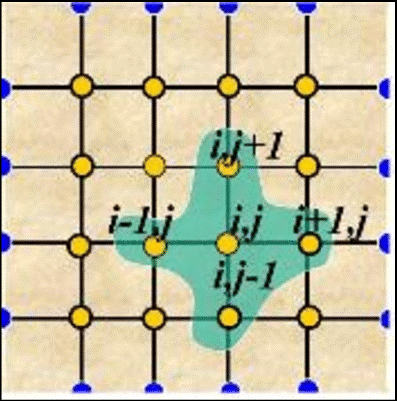Figure 2. Gridiron typography of finite difference

with the boundary conditions,

$u_{0}=h\left(\frac{\partial u_{0}}{\partial y}\right), \quad u_{1}=h\left(\frac{\partial u_{1}}{\partial y}\right), \quad \theta_{0}=1, \quad \theta_{1}=1$

$\phi_{0}=1, \phi_{1}=1,$ at $y=0$                          (33)

$u_{0} \rightarrow 0, \quad u_{1} \rightarrow 0, \quad \theta_{0} \rightarrow 1, \quad \theta_{1} \rightarrow 1$

$\phi_{0} \rightarrow 1, \quad \phi_{1} \rightarrow 1 \quad$ as $y \rightarrow \infty$

Now the Eqns. (29)-(33) are transformed into the following finite difference form.

$\frac{v_{i, j}-v_{i, j-1}}{\Delta y}=0$       (34)

$\frac{u_{i, j}^{\prime}-u_{i, j}}{\Delta t}+v_{i, j}\left(\frac{u_{i, j+1}-u_{i, j}}{\Delta y}\right)=\left(\frac{u_{i, j+1}-2 u_{i, j}+u_{i, j-1}}{(\Delta y)^{2}}\right)+G r \theta_{i, j}+G c \phi_{i, j}-\left(M+\frac{1}{k}\right) u_{i, j}$       (35)

$\frac{\theta_{i, j}-\theta_{i, j}^{\prime}}{\Delta t}+v_{i, j}\left(\frac{\theta_{i, j+1}-\theta_{i, j}}{\Delta y}\right)=\frac{1}{\operatorname{Pr}}\left(1+\frac{4}{3} R\right)\left(\frac{\theta_{i, j+1}-2 \theta_{i, j}+\theta_{i, j-1}}{(\Delta y)^{2}}\right)$      (36)

$\frac{\phi_{i, j}^{\prime}-\phi_{i, j}}{\Delta t}+v_{i, j}\left(\frac{\phi_{i, j+1}-\phi_{i, j}}{\Delta y}\right)=\left(\frac{1}{S c}\right)\left(\frac{\phi_{i, j+1}-2 \phi_{i, j}+\phi_{i, j-1}}{(\Delta y)^{2}}\right)-K r(\phi)$    (37)

Here boundary conditions for the obtained equations become

$u_{i, j}^{0}=h\left(\frac{u_{i, j+1}-u_{i, j}}{\Delta y}\right), \theta_{i, j}^{0}=\left(1+\varepsilon e^{i w t}\right)$

$\phi_{i, j}^{0}=1+\varepsilon e^{i w t} \quad$ at $\quad y \rightarrow 0$

$u_{i, j}^{n}=0, \quad \theta_{i, j}^{n}=0$                                    (38)

$\phi_{i, j}^{n}=0 \quad$ as $\quad y \rightarrow \infty$

Here i j denote the mesh point M=100 and N=200 with X, Y coordinates respectively, but the superscripts n represents a value of time, t=n∆t where n=[0, ∞].

Figures 16-19 that have been obtained for equations of finite difference method are drawn for different values of t. It is obtained that for different values of t, which are taken from 0.05 to 1.00 and the value of ∆t=0.00005.

The stability condition for this work has been established as [2, 15],

$\frac{4}{\operatorname{Pr}}\left(1+\frac{4}{3} R\right) \frac{\Delta t}{(\Delta y)^{2}}+|-v| \frac{\Delta t}{\Delta y} \leq 1$ and

$\left(\frac{1}{S c}\right) \frac{2 \Delta t}{(\Delta y)^{2}}+|-v| \frac{\Delta t}{\Delta y}-\Delta t k r \leq 1$        (39)

With initial boundary conditions, u=0, v=0, θ=0, ϕ=0 at τ=0 and for ∆t=0.0005 and ∆Y=0.20 then the problem will be converged at Pr≥0.117 and Sc≥0.025.

5. Results and Discussion

The composite framework of differential equations with boundary conditions is shown graphically by utilizing Compaq Visual Fortran and tecplot 9. The values of the parameters are considered for our investigation are, Gr=5.0, Gc= 5.0, R=1.0, wt=π/2, Sc=0.60, K=1.0, Pr=0.71, Kr=0.6, M=1.0, A=0.5, h=0.1, Ɛ=0.001. However, Table 1 represents the validation of the current work. It has been noticed that the impression of Prandtl number, chemical reaction, Schmidt number, thermal and mass Grashof number have an identical impact on the respective fluid flow. However, for the case of the radiation parameter, different results have been observed in the temperature profiles. Figure 3 portrays the impacts of thermal Grashof number on velocity profile. Because of improvement in buoyance force, it is discovered that an addition in the thermal Grashof number and raise the velocity field. The comparing positive estimations of the thermal Grashof number demonstrate the cooling of the surface. It is shown that the mathematical values in the numerical solutions are picked up as, 1.84562, 2.45874, 3.15643, 3.73257 for Gr=5.0, 10.0, 15.0, 20.0 individually at y=0.84535. Figure 3 demonstrates that the velocity increments 75.06%, 77.88%, 84.56% due to Gr=5 to Gr=10, Gr=10 to Gr=15, and Gr=15 to Gr=20, respectively thermal Grashof number is the proportion of the species lightness power to the full hydrodynamic power. The velocity lays in the boundary layer for the effect of modified Grashof number and illustrates in Figure 4. It is seen that the numerical values in the numerical solutions are found as 2.53247, 3.72613, 5.15284, 6.35522 for Gc = 5.0, 10.0, 15.0, 20.0 separately at y = 0.83136.

Table 1. Graphical comparison of presents outcomes with previous outcomes 

 Parameter Previous results given Arifuzzaman et al.  Presents results U θ ϕ θ ϕ U Pr Dec Dec R Inc Dec Kr Dec Dec Sc Dec Dec Gr Inc Inc Gc Inc Inc

Inc- Increase; Dec- Decrease

## 3.pngFigure 3. Effects of Gr on velocity distribution

## 4.pngFigure 4. Effects of Gc on velocity distribution

The highest velocity values for different values of Gc is marked with a red circle. Figure 4 demonstrates that the velocity increments 68.06%, 72.32%, 81.07% because of Gc =5 to Gc=10, Gc=10 to Gc=15 and Gc=15 to Gc=20. Impact of magnetic parameter (M) on the velocity of fluid flow has been shown in Figure 5. In this figure, there are four various velocity profiles are drawn for the four distinct estimations of magnetic parameter (M). It is shown that the velocity profiles decreases for the effect of magnetic parameter for the reason of Lorentz force. In Figure 6 illustrates the velocity profiles with the effect of permeable medium parameter (K). In the figure, distinctive estimations of K are taken for the diverse lines of the chart. It is seen that there exist four lines for the four distinct estimations of K and increases for the raise of K. Here the numerical values in the numerical solutions are picked up from the figure as 1.84518, 2.13452, 2.42367, 2.67352 for K=1.00, 2.00, 3.00, 4.00 individually at y=0.73179. Figure 6 demonstrates that the velocity increments 86.45%, 88.07%, 90.64% due to K=1.00 to K=2.00, K=2.00 to K=3.00, and K=3.00 to K=4.00.

## 5.png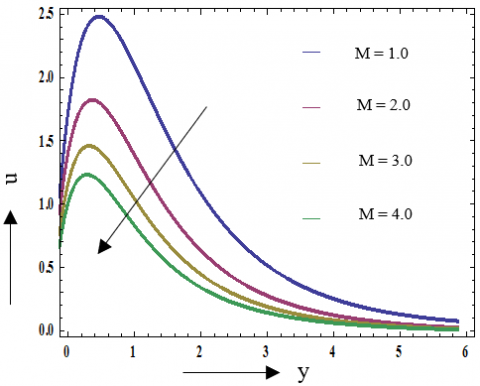Figure 5. Effects of M on velocity distribution

## 6.pngFigure 6. Effects of K on velocity distribution

Figure 7 illustrates the velocity profile alongside the impacts of radiation parameter (R). For each estimation of R, the velocity profiles increase. It is seen that the numerical values in the numerical solution appear 2.51538, 3.03753, 3.22381, 3.47352 for R=1.00, 2.00, 3.00, 4.00 separately at y=0.63179. Figure 7 demonstrates that the velocity increments 82.81%, 94.22%, 92.71% due to R=1.00 to R=2.00, R=2.00 to R=3.00 and R=3.00 to R=4.00. Impacts of Prandtl number on velocity profiles appear in Figure 8.

## 7.png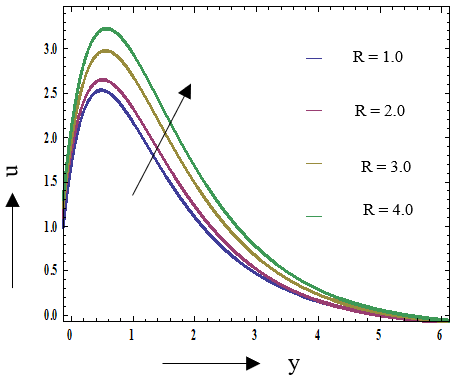Figure 7. Effects of R on velocity distribution

## 8.pngFigure 8. Effects of Pr on velocity distribution

## 9.png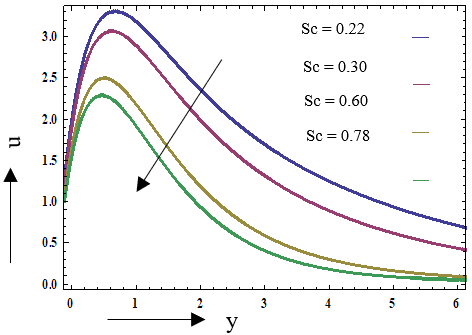Figure 9. Effects of Schmidt number Sc on velocity

For various estimations of Prandtl number, it is seen that there exist diverse lines which show the connection of velocity profile and Pr. From the figure, it very well may be said that the estimation of velocity profiles are likewise contrarily relative alongside the estimation of Pr. Additionally, the numerical values in the numerical solutions are found as 2.54248, 2.23423, 1.42381, 1.37452 for Pr=0.71, 1.0, 3.0, 7.0 individually at y=0.73379. In Figure 9 the relation between Schmidt number and velocity profiles. With different values of Schmidt number are taken for y and have different lines that represent velocity profiles. In this figure, we see that the lines go downstairs for higher values of Sc. It is cleared that the numerical values in the numerical solutions are picked up as 3.24228, 3.03313, 2.42271, 2.27452 for Sc= 0.22, 0.30, 0.60, 0.78 separately at y=0.83279. Figure 9 demonstrates that the velocity diminishes 93.51%, 79.86%, 93.80% for Sc=0.22 to Sc=0.30, Sc=0.30 to Sc=0.60 and Sc=0.60 to Sc=0.78.

Impacts of a chemical reaction (Kr) on the velocity of the flow field appear in Figure 10. The lines have given the connection between velocity profiles and the chemical reaction parameter. With the increment of Kr, velocity profiles diminish. It very well may be said from Figure 10 that the numerical values in the numerical solutions are picked up as 2.19373, 2.09781, 1.97753, 1.89923 due to Kr=0.60, 1.00, 1.60, 2.00 individually at y=0.79872. Figure 10 demonstrates that the velocity diminishes 95.43%, 94.25%, 95.93% for Kr=0.60 to Kr=1.00, Kr=1.00 to Kr=1.60 and Kr=1.60 to Kr=2.00.

## 10.pngFigure 10. Effects of Kr on velocity distribution

## 11.png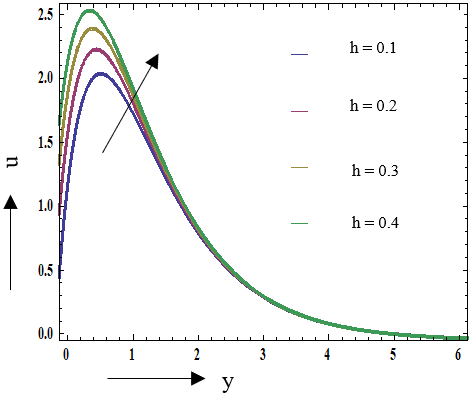Figure 11. Effects of h on velocity distribution

Figure 11 shows the connection between velocity profiles and the refraction parameter (h). With the increment of h, the velocity profiles increase. Here the numerical values in the numerical solutions are appeared 2.07458, 2.28493, 2.43361, 2.57422 for h=0.1, 0.2, 0.3, 0.4 individually at y=0.60019. Figure 10 demonstrates that, velocity increments 90.78%, 93.82%, 94.55% due to h=0.1 to h=0.2, h=0.2 to h=0.3 and h=0.3 to h=0.4. Figure 12 is for the impacts of a suction parameter (A) on velocity profiles. Here the curves decrease, which means for the highest value of A, the value of velocity will be the minimum, and for a minimum value of A velocity will be maximum. Observing Figure 12, the numerical values in the numerical solution is obtained as 2.24258, 2.19923, 2.18881, 1.16742 for A=5, 10, 50, 100, respectively, at y=0.63379. Figure 12 shows that the velocity decreases 36.48%, 49.85%, 10.36% due to A=5 to A=10, A=10 to A=50 and A=50 to A=100.

## 12.png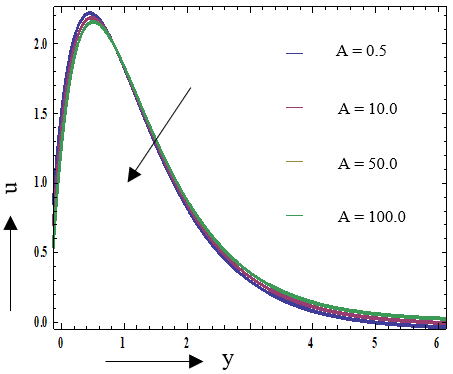Figure 12. Effects of A on velocity distribution

## 13.pngFigure 13. Effects of Pr on temperature distribution

In Figure 13, the curves represent the temperature profiles with the change of Prandtl number (Pr). Temperature profiles decrease with the increases of Pr. The numerical values are showed as 0.254248, 0.18423, 0.10381, 0.07452 for Pr=0.71, 1.00, 3.00, 7.00 respectively at y=0.12437, 0.27546, 1.00342, 1.32146. Figure 13 also shows that the velocity decreases 72.01%, 55.55%, 70.36% due to Pr=0.71 to Pr=1.00, Pr=1.00 to Pr=3.00 and Pr=3.00 to Pr=7.00. For a small variation in Prandtl number, the thermal conductivity and temperature can be distributed from the temperature plate more rapidly than the Prandtl number.

Figure 14 shows temperature profiles with the increment of radiation parameter. This speaks to that the connection between radiation number and temperature profiles are contrarily relative. So that, the temperature profiles esteem will be expanded as radiation number rise. The computing numerical values are picked up as 0.24248, 0.22423, 0.19381, 0.11452 for R=1.00, 2.00, 3.00, 4.00 separately at y=1.21345, 1.73379, 1.98451, 2.00932. Figure 14 demonstrates that the velocity diminishes 91.66%, 86.36%, 57.89% for R=0.71 to R=1.00, R=1.00 to R=3.00 and R=3.00 to R=4.00. The temperature profile chart changes their belongings as well as raises the thickness of the thermal in the boundary layer.

## 14.png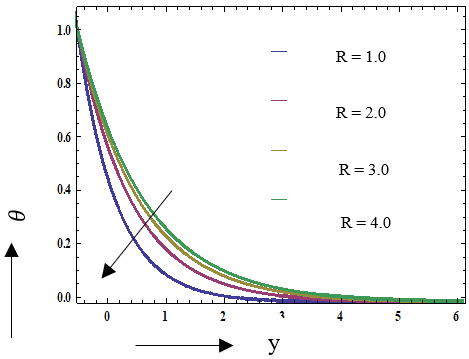Figure 14. Effects of R on temperature distribution

## 15.png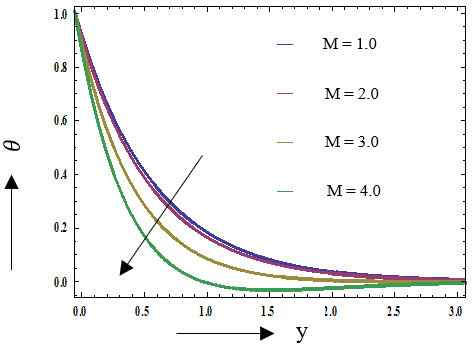Figure 15. Effects of M on temperature distribution

## 16.png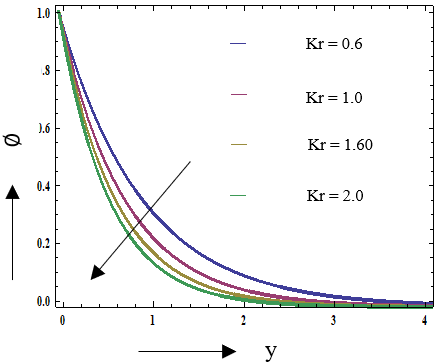Figure 16. Effects of Kr on concentration distribution

Figure 15 is depicted for different values of the magnetic parameter on temperature distribution. It is evident from Figure 15 that the temperature decreases with the increase of the magnetic parameter because the magnetic field produces Lorentz force, which is the cause of temperature decrement. Figure 16 represents the concentration profiles alongside the chemical reaction parameter (Kr). Four bends that appeared in Figure 16 shows four diverse concentration profiles for four distinct estimations of the synthetic response parameter. The bend got for high estimation of Kr is lower than the bend got for low concoction response esteem. When the concoction response esteem went high, focus profiles will be lower, and for low estimation of Kr, at that point, concentration esteem will be high.

## 17.png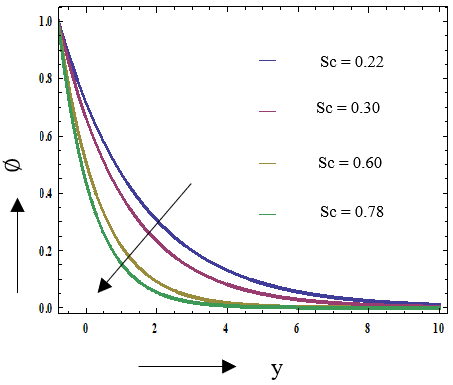Figure 17. Effects of Sc on concentration distribution

## 18.png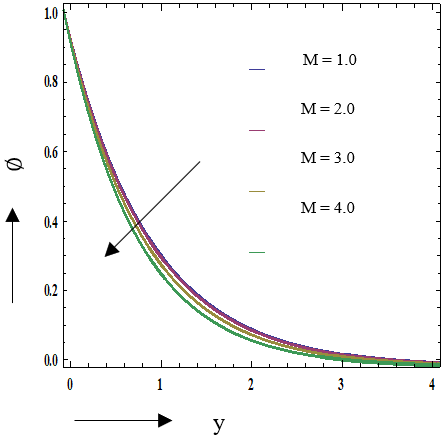Figure 18. Effects of M on concentration distribution

It is seen that the numerical values in the numerical solutions are picked up as 0.19248, 0.17123, 0.12381, 0.09952 for Kr=0.60, 1.0, 1.60, 2.0 separately at y=1.73379, 1.89905, 2.00165, 2.20134. Figure 16 demonstrates that the velocity diminishes 89.28%, 70.58%, 75.06% for Kr=0.60 to Kr=1.0, Kr=1.0 to Kr=1.60 and Kr=1.60 to Kr=2.0.

Figure 17 shows the concentration profile for different values of the Schmidt number. For an increasing value of the Schmidt number, the concentration graph decreases. The impact of the magnetic parameter (M) on concentration distribution is depicted in Figure 18. It is noticed that from Figure 18, the concentration decreases for the increment of the magnetic parameter because the magnetic field has a thickening effect on the thermal boundary layer, which gives a decrease in the concentration.

## 19.pngFigure 19. Effects of M on skin friction profile

## 20.png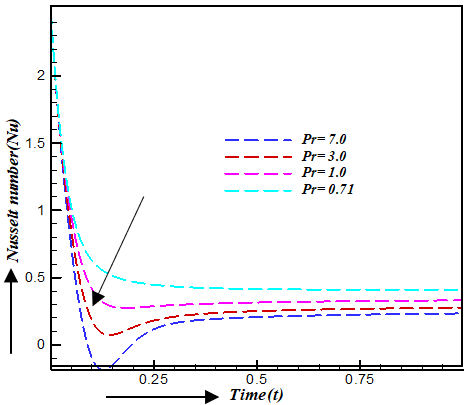Figure 20. Effects of Pr on Nu

## 21.png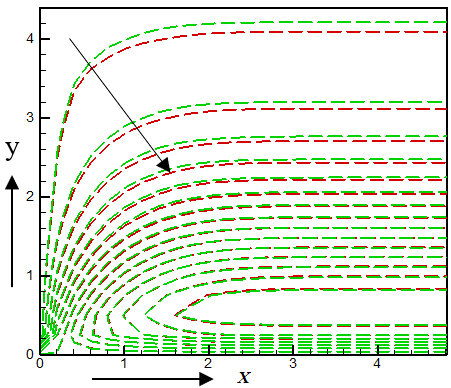Figure 21. Streamlines when Pr=1.8(Red dashed line) and Pr=1.2(Green line)

The skin friction and Nusselt number profiles are depicted in Figure 19 and 20, respectively. Skin friction profile is shown for different values of magnetic parameter (M). It is examined from a figure that skin friction is low for large values of the magnetic parameter. Nusselt number profile is depicted for various values of Prandtl number, and the Nusselt number profile decreases for higher values of Prandtl number. Figure 21 shows the streamlines (line view) for different values of Pr, and Figure 22 shows the streamlines (flood view) for different values of Pr. And the Streamlines shows the decreasing of fluid flow velocity with the increment of Pr. Figure 23 and 24 shows the isotherm lines for different values of radiation parameter R. For the increment of R, the isotherm lines increases.

## 22.png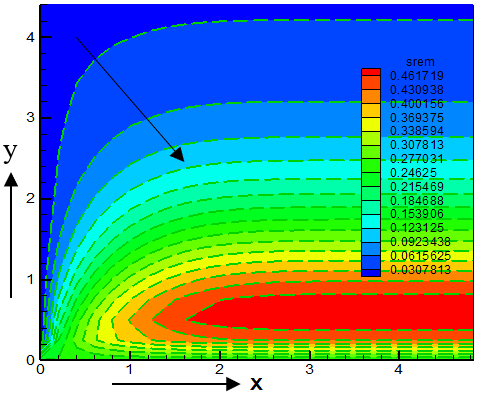Figure 22. Streamlines flood view when Pr=1.8 and Pr=1.2

## 23.png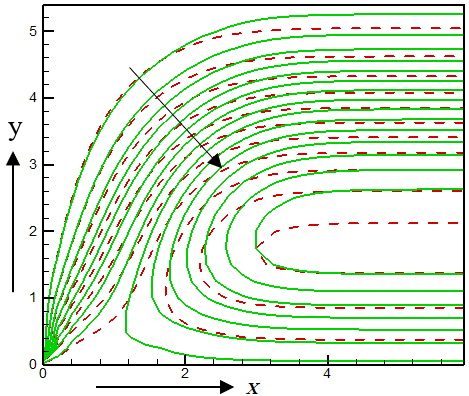Figure 23. Isotherms when R=2.5 (Red dashed line) and R=2.0 (Green line)

## 24.pngFigure 24. Isotherms flood view when R=2.5 and R=2.0

6. Conclusions

Briefly, the outcomes of the present investigation can be described below:

• The developing values of Grashof number, refraction parameter, modified Grashof number, and permeability parameter help to upsurge the velocity field while the pertinent parameters like Prandtl number, radiation parameter, chemical reaction parameter, Schmidt number, and suction parameter have decreasing tendency on the same profile.
• The fluid temperature diminishes with an increment in the Prandtl number, radiation parameter, and magnetic parameter
• The concentration profiles represent declining behavior for the expansion of chemical reaction parameter Schmidt number and magnetic parameter.
• However, the Nusselt number and skin friction profiles have illustrated decreasing behavior for the upsurging values of the Prandtl number and magnetic parameter.

Moreover, both the Prandtl number and radiation parameters have shown the plunging attitude in both streamlines and isothermal lines.

Nomenclature
 A suction parameter, (-) B0 magnetic component, (Wbm-2) $\bar{C}$ species concentration in the boundary layer, (-) Cf skin friction, (-) $\bar{C}_w$ concentration of the plate, (-) $\bar{C}_{\infty}$ concentration far away from the plate, (-) Cp specific temperature at constant pressure, (Jkg-1K-1) D chemical molecular diffusivity, (-) g acceleration due to gravity, (ms-2) Gr thermal Grashof number, (-) Gc modified Grashof number, (-) h refraction parameter, (-) $\bar{K}$ permeability parameter, (-) K dimensionless permeability parameter, (-) Kr chemical reaction number, (-) M magnetic parameter, (-) Pr Prandtl number, (-) R radiation parameter, (-) Sc Schmidt number, (-) $\bar{T}$ temperature in the boundary layer, (k) $\bar{T}_w$ temperature of the plate, (k) $\bar{T}_{\infty}$ temperature far away from the plate, (k) u,v dimensionless velocity $\bar{u}, \bar{v}$ dimensional velocity $\bar{w}$ frequency of oscillation, (-) w dimensionless frequency of oscillation (-) $\bar{x}, \bar{y}$ coordinate axes along the plate x,y dimensionless coordinate Greek symbols α the fluid thermal diffusivity β volumetric coefficient of thermal expansion $\bar{\beta}$ volumetric concentration expansion coefficient σ electric conductivity of the fluid k thermal conductivity, (Wm-1K-1) ρ density of the fluid, (kgm-3) v kinematic viscosity, (m2s-1) θ dimensionless temperature, (-) ϕ dimensionless concentration, (-) ε variation parameter, (-) Abbreviation MHD magnetohydrodynamic
References

 Malapati, V., Polarapu, P. (2015). Unsteady MHD free convective heat and mass transfer in a boundary layer flow past a vertical permeable plate with thermal radiation and chemical reaction. Procedia Engineering, 127: 791-799. https://doi.org/10.1016/j.proeng.2015.11.414

 Arifuzzaman, S.M., Rana, B.J., Ahmed, R., Ahmmed, S. F. (2017). Cross diffusion and MHD effects on a high order chemically reactive micropolar fluid of naturally convective heat and mass transfer past through an infinite vertical porous medium with a constant heat sink. In AIP Conference Proceedings, 1851(1): 020006. https://doi.org/10.1063/1.4984635

 Das, S., Jana, R.N., Chamkha, A.J. (2015). Unsteady free convection flow past a vertical plate with heat and mass fluxes in the presence of thermal radiation. Journal of Applied Fluid Mechanics, 8(4): 845-854. https://doi.org/10.18869/acadpub.jafm.73.238.23265

 Arifuzzaman, S.M., Khan, M.S., Islam, M.S., Islam, M.M., Rana, B.M.J., Biswas, P., Ahmmed, S.F. (2017). MHD Maxwell fluid flow in presence of nano-particle through a vertical porous–plate with heat-generation, radiation absorption and chemical reaction, front. Heat Mass Transfer, 9: 1-14. https://doi.org/10.5098/hmt.9.25

 Moalem, D. (1976). Steady state heat transfer within porous medium with temperature dependent heat generation. International Journal of Heat and Mass Transfer, 19(5): 529-537. https://doi.org/10.1016/0017-9310(76)90166-6

 Das, U.N., Deka, R., Soundalgekar, V.M. (1994). Effects of mass transfer on flow past an impulsively started infinite vertical plate with constant heat flux and chemical reaction. Forschung im Ingenieurwesen, 60(10): 284-287. https://doi.org/10.1007/BF02601318

 Anjalidevi, S.P., Kandasamy, R. (1999). Effects of chemical reaction, temperature and mass transfer on laminar flow along a semi-infinite horizontal plate. Temperature and Mass Transfer, 35(6): 465-467. https://doi.org/10.1007/s002310050349

 Arifuzzaman, S.M., Hossain, K.E., Roy, R., Islam, M., Akter, S., Khan, M. (2017). Chemically reactive viscoelastic fluid flow in presence of nano particle through porous stretching sheet. Frontiers in Heat and Mass Transfer (FHMT), 9(1). https://doi.org/10.5098/hmt.9.5

 Chamkha, A.J., Ahmed, S.E. (2012). Unsteady MHD heat and mass transfer by mixed convection flow in the forward stagnation region of a rotating sphere at different wall conditions. Chemical Engineering Communications, 199(1): 122-141. https://doi.org/10.1080/00986445.2011.575907

 Bhuvanavijaya, R., Mallikarjuna, B. (2014). Effect of variable thermal conductivity on convective temperature and mass transfer over a vertical plate in a rotating system with variable porosity regime. Journal of Naval Architecture and Marine Engineering, 11(1): 83-92. http://dx.doi.org/10.3329/jname.v11i1.16488

 Arifuzzaman, S.M., Khan, M.S., Mehedi, M.F.U., Rana, B.M.J., Ahmmed, S.F. (2018). Chemically reactive and naturally convective high speed MHD fluid flow through an oscillatory vertical porous plate with heat and radiation absorption effect. Engineering Science and Technology, an International Journal, 21(2): 215-228. https://doi.org/10.1016/j.jestch.2018.03.004

 Chaudhary, R.C., Jain, P. (2006). Hall effect on MHD mixed convection flow of a viscoelastic fluid past an infinite vertical porous plate with mass transfer and radiation. Theoretical and Applied Mechanics, 33(4): 281-309. https://doi.org/10.2298/TAM0604281C

 Kumar, R., Chand, K. (2011). Effect of slip condition and Hall current on unsteady magneto hydrodynamics (MHD) flow of viscoelastic fluid past an infinite vertical porous plate through porous medium. International Journal of Engineering Science and Technology, 3(4): 3124-3133. https://doi.org/10.34893/5YTNJ9F8

 Arifuzzaman, S.M., Mehedi, M.F.U., Mamun, A.A., Biswas, P., Islam, M.R., Khan, M.S. (2018). Micropolar fluid flow in presence of nanoparticles. International Journal of Temperature and Technology, 36(3): 936-948. https://doi.org/10.18280/ijht.360321

 Nield, D.A., Bejan, A. (2017). Convection in Porous Media. Switzerland. 2nd Edition, Springer-Verlag, Berlin.

 Zueco, J., Ahmed, S. (2010). Combined heat and mass transfer by mixed convection MHD flow along a porous plate with chemical reaction in presence of heat source. Applied Mathematics and Mechanics, 31(10): 1217-1230. https://doi.org/10.1007/s10483-010-1355-6

 Sahoo, S.N., Panda, J.P., Dash, G.C. (2011). Unsteady two-dimensional magneto hydrodynamic (MHD) flow and temperature transfer of an elastic viscous liquid past an infinite hot vertical porous surface. A.M.S.E France, 80(2): 26-42.

 Mamun, A.A., Arifuzzaman, S.M., Reza-E-Rabbi, S., Biswas, P., Khan, M.S. (2019). MHD effects on radiative sisko nanofluids through a nonlinearly stretching sheet. International Journal of Temperature and Technology, 37(1): 285-295. https://doi.org/10.18280/ijht.370134

 Al-Mudhaf, A., Chamkha, A.J. (2005). Similarity solutions for magneto hydrodynamic (MHD) thermo modified Marangoni convection over a flat surface in the presence of temperature generation or absorption effects. Temperature and Mass Transfer, 42(2): 112-121. https://doi.org/10.1007/s00231-004-0611-8

 Arifuzzaman, S.M., Khan, M.S., Mamun, A.A., Reza-E-Rabbi, Sk., Biswas, P., Ifsana Karim (2018). Hydrodynamic stability on MHD radiative fourth-grade fluid through porous plate. Journal of King Saud University, 1018-3647 https://doi.org/10.1016/j.jksus.2018.12.009 1018-3647

 Narahari, M., Dutta, B.K. (2012). Effects of thermal radiation and mass diffusion on free convection flow near a vertical plate with Newtonian temperatureing. Chemical Engineering Communication, 199(5): 628-643. https://doi.org/10.1080/00986445.2011.611058

 Sharma, P.R., Singh, G. (2008). Unsteady magneto hydrodynamic (MHD) free convective flow and temperature transfer along a vertical porous plate with variable suction and internal temperature generation. International Journal of Applied Mathematics and Mechanics, 4(5): 1-8.

 Arifuzzaman, S.M., Biswas, P., Mehedi, M., Uddin, F., Al-Mamun, A., Ahmmed, S.F., Khan, M.S. (2018). Analysis of unsteady boundary layer viscoelastic nanofluid flow through a vertical porous plate with thermal radiation and periodic magnetic field. Journal of Nanofluids, 7(6): 1122-1129. https://doi.org/10.1166/jon.2018.1547

 Mostafa, M.A. (2007). The variable viscosity and chemical reaction effects on mixed convection temperature and mass transfer along a semi-infinite vertical plate. Journal of Mathematical Problems and Engineering, 2007: 41323. https://doi.org/10.1155/2007/41323

 Biswas, P., Arifuzzaman, S.M., Karim, I., Khan, M. (2017). Impacts of magnetic field and radiation absorption on mixed convective Jeffrey Nano fluid flow over a vertical stretching sheet with stability and convergence analysis. Journal of nanofluids, 6(6): 1082-1095. https://doi.org/10.1166/jon.2017.1407

 Dursunkaya, Z., Worek, W.M. (1992). Diffusion-thermo and thermal-diffusion effects in transient and steady natural convection from a vertical surface. International Journal of Heat and Mass Transfer, 35(8): 2060-2065. https://doi.org/10.1016/0017-9310(92)90208-A

 Biswas, P., Arifuzzaman, S.M., Rahman, M., Khan, M.S. (2018). Effects of periodic magnetic field on 2D transient optically dense gray nanofluid over a vertical plate: a computational EFDM study with SCA. Journal of Nanofluids, 7(1): 82-91. https://doi.org/10.1166/jon.2018.1434

 Rahman, M.M., Sattar, M.A. (2006). Magneto hydrodynamic convective flow of a micro polar fluid past continuously moving vertical porous plate in the presence of temperature generation or absorption. Journal of Temperature Transfer, 128(2): 142-152. https://doi.org/10.1115/1.2136918

 Makinde, O.D. (2011). Similarity solution of hydro magnetic parameter temperature and mass transfer over a vertical plate with internal generation and a convective boundary condition. Thermal Science, 15(1): S137-S143. http://www.academicjournals.org/IJPS

 Khan, M., Karim, I., Rahman, M., Arifuzzaman, S.M., Biswas, P. (2017). Williamson fluid flow behaviour of MHD convective-radiative Cattaneo–Christov heat flux type over a linearly stretched-surface with heat generation and thermal-diffusion. Frontiers in Heat and Mass Transfer (FHMT), 9(1). http://dx.doi.org/10.5098/hmt.9.15

 Makinde, O.D. (2010). Similarity solution of hydromagnetic heat and mass transfer over a vertical plate with a convective surface boundary condition. International Journal of the Physical Sciences, 5(6): 700-710.

 Wendt, J.F. (2008). Computational Fluid Dynamics: An Introduction. Springer Science & Business Media.

 Bakr, A.A. (2011). Effects of chemical reaction on MHD free convection and mass transfer flow of micro polar fluid with oscillatory pate velocity and constant temperature source in a rotating frame of reference. Communication in Nonlinear Science and Numerical Simulation, 16(2): 698-710. https://doi.org/10.1016/j.cnsns.2010.04.040

 Mahdy, A. (2010). Effect of chemical reaction and temperature generation or absorption on double-diffusive convection from a vertical truncated cone in porous media with variable viscosity. International Communication in Temperature and Mass Transfer, 37(5): 548-554. https://doi.org/10.1016/j.icheatmasstransfer.2010.01.007

 Pal, D., Mondal, H. (2012). Soret and Dufour effects on MHD non-Darcian mixed convection heat and mass transfer over a stretching sheet with non-uniform heat source/sink. Physica B: Condensed Matter, 407(4): 642-651. https://doi.org/10.1016/j.physb.2011.11.051

 Mondal, R.N., Islam, S., Uddin, K., Hossain, A. (2013). Effects of aspect ratio on unsteady solutions through curved duct flow. Applied Mathematics and Mechanics, 34(9): 1107-1122. https://doi.org/10.1007/s10483-013-1731-8